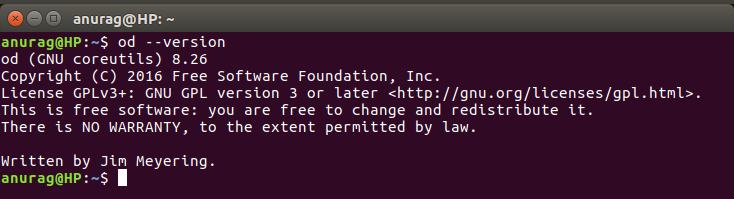Open In App

# od command in Linux with example

od command in Linux is used to convert the content of input in different formats with octal format as the default format.This command is especially useful when debugging Linux scripts for unwanted changes or characters. If more than one file is specified, od command concatenates them in the listed order to form the input.It can display output in a variety of other formats, including hexadecimal, decimal, and ASCII. It is useful for visualizing data that is not in a human-readable format, like the executable code of a program.

Syntax :

```od [OPTION]... [FILE]...
```

Options of od command

1. -b Option :It displays the contents of input in octal format.

SYNTAX :

```\$ od -b input.txt
```

EXAMPLE :

```input :
100
101
102
103
104
105
```

OUTPUT :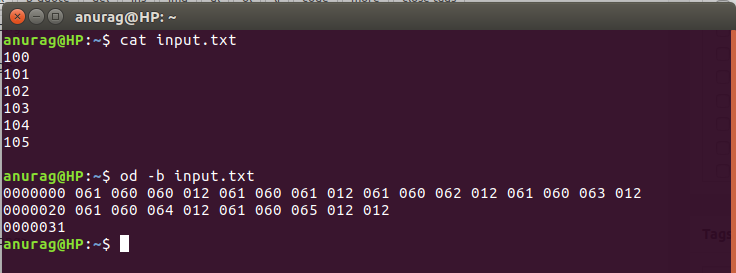The first column in the output of od represents the byte offset in file.

2. -c Option :It displays the contents of input in character format.

SYNTAX :

```\$ od -c input.txt
```

EXAMPLE :

```input :
100
101
102
103
104
105
```

OUTPUT :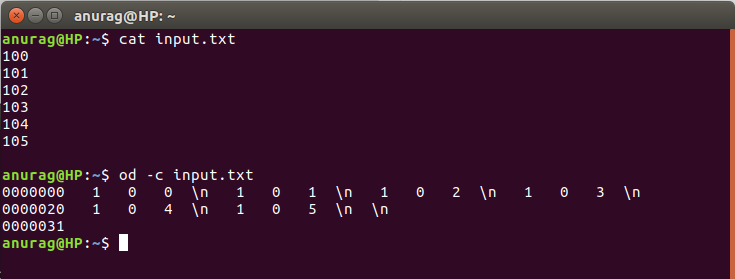3. -An Option :It displays the contents of input in character format but with no offset information.

SYNTAX :

```\$ od -An -c input.txt
```

EXAMPLE :

```input :
100
101
102
103
104
105
```

OUTPUT :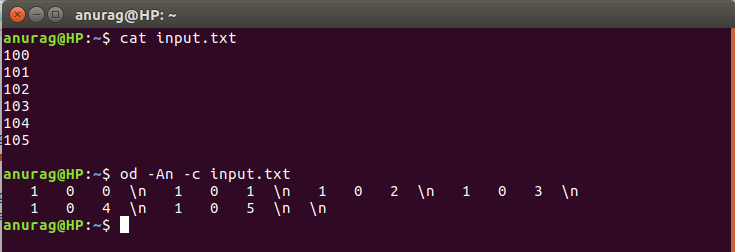4. -A Option :It displays the contents of input in different format by concatenation some special character with -A.
For example:
1. -Ax for Hexadecimal format(we concatenate x with -A)
2. -Ao for Hexadecimal format(we concatenate o with -A)

SYNTAX :

```\$ od -Ax input.txt
\$ od -Ao input.txt
```

EXAMPLE :

```input :
100
101
102
103
104
105
```

OUTPUT :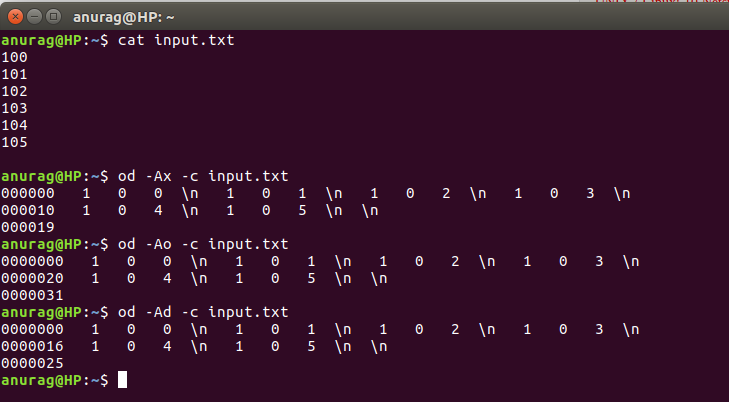5. – Option :Accept input from command line.

SYNTAX :

``` \$ od -c -
```

EXAMPLE :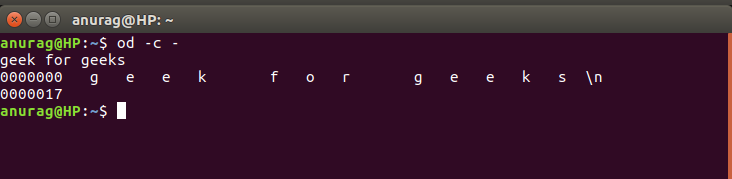Here, we see that first the input was given and then after pressing the ctrl+d the od command output was displayed.

6. Display hidden character in a file :

Consider the following content of file :

`Geek ^Mforgeeks`

If a file containing the above string is printed using the cat command, following output is seen :

```
\$ cat file
\$ forgeekseek
```

so,in order to remove it we use,

OUTPUT :

```\$ od -c file
0000000   G   e   e    k       f    o     r        \r   g    e    e    k    s  \n
0000020
```

7. -j Option :It displays the output after skipping some bytes.

SYNTAX :

```\$ od -j4 -c input.txt
```

EXAMPLE :

```input :
100
101
102
103
104
105
```

OUTPUT :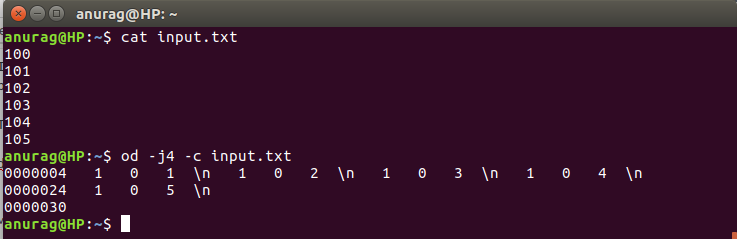Here,initial 4 bytes were skipped from output.

8. -N Option :It display limited bytes in output using -N option.

SYNTAX :

```\$ od -N4 -c input.txt
```

EXAMPLE :

```input :
100
101
102
103
104
105
```

OUTPUT :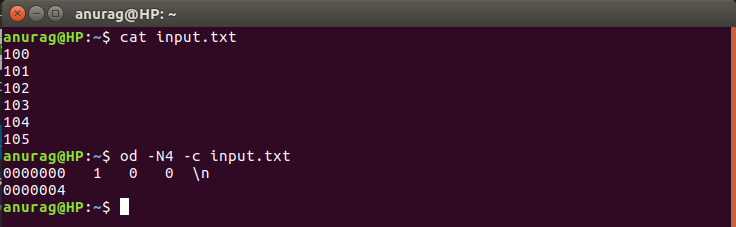Here,initial 4 bytes were displayed from output.It is the opposite of -j option.

9. -w Option :It is used to customize the output width.

SYNTAX :

```\$ \$ od -w1 -c -Ad input.txt
```

EXAMPLE :

```input :
100
101
102
103
104
105
```

OUTPUT :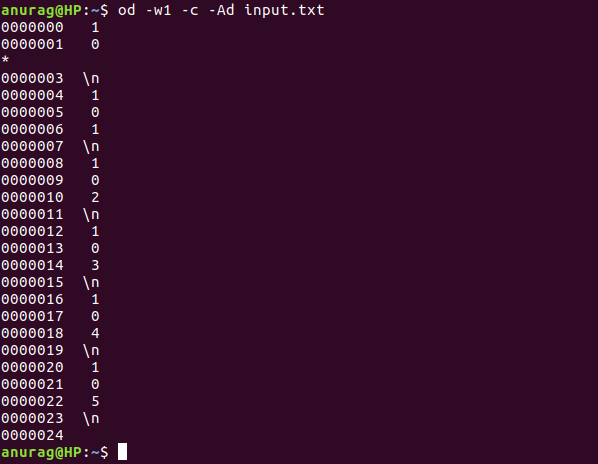So we see that output width was reduced to 1

10. -v Option :It is used to output duplicate values.As can be observed in the output above, a * was printed. This is done to suppress the output of lines that are same or duplicates. But through -v option these lines can also be printed.

SYNTAX :

```\$ \$ od -w1 -v -c -Ad input.txt
```

EXAMPLE :

```input :
100
101
102
103
104
105
```

OUTPUT :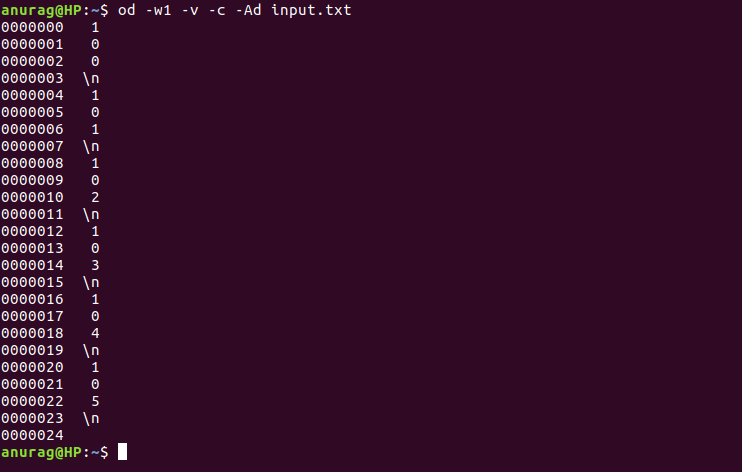11. -i Option :It display output as decimal integer.

SYNTAX :

```\$ \$ od -i input.txt
```

EXAMPLE :

```input :
100
101
102
103
104
105
```

OUTPUT :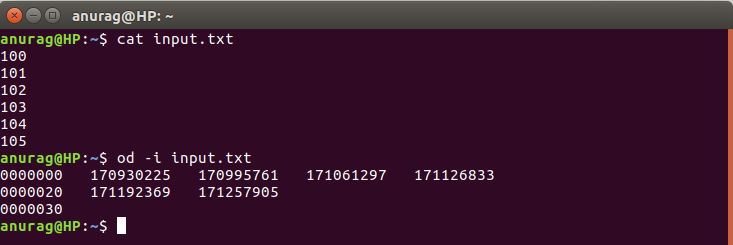12. -o Option :It display output as octal 2 byte units.

SYNTAX :

```\$ \$ od -o input.txt
```

EXAMPLE :

```input :
100
101
102
103
104
105
```

OUTPUT :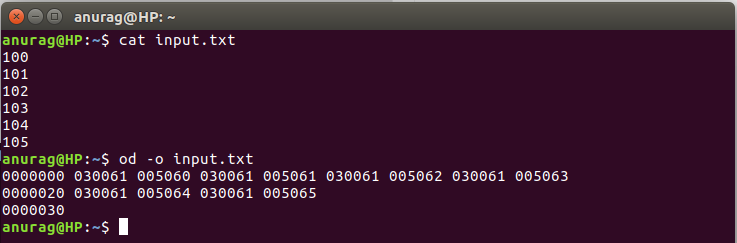13. -x Option :It display output as hexadecimal 2 byte units

SYNTAX :

```\$ \$ od -x input.txt
```

EXAMPLE :

```input :
100
101
102
103
104
105
```

OUTPUT :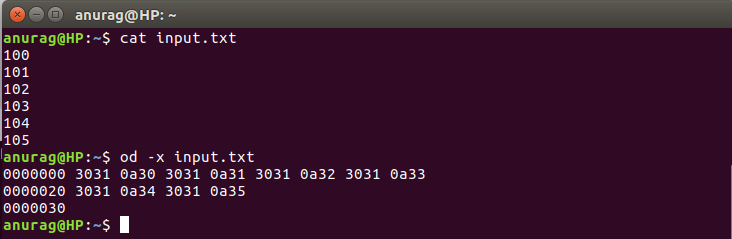14. -t Option : It select output format and display it.
Traditional format specifications may be intermixed; they accumulate:
-a same as -t a, select named characters, ignoring high-order bit
-b same as -t o1, select octal bytes
-c same as -t c, select printable characters or backslash escapes
-d same as -t u2, select unsigned decimal 2-byte units
-f same as -t fF, select floats
-i same as -t dI, select decimal ints
-l same as -t dL, select decimal longs
-o same as -t o2, select octal 2-byte units
-s same as -t d2, select decimal 2-byte units
-x same as -t x2, select hexadecimal 2-byte units

SYNTAX :

```\$ \$ od -ta input.txt
```

EXAMPLE :

```input :
100
101
102
103
104
105
```

OUTPUT :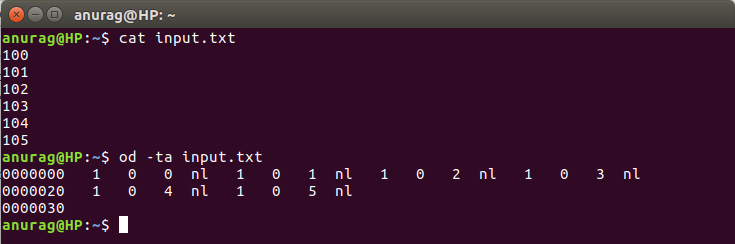15. –help Option :It displays help information.

SYNTAX :

```\$ \$ od --help
```

OUTPUT :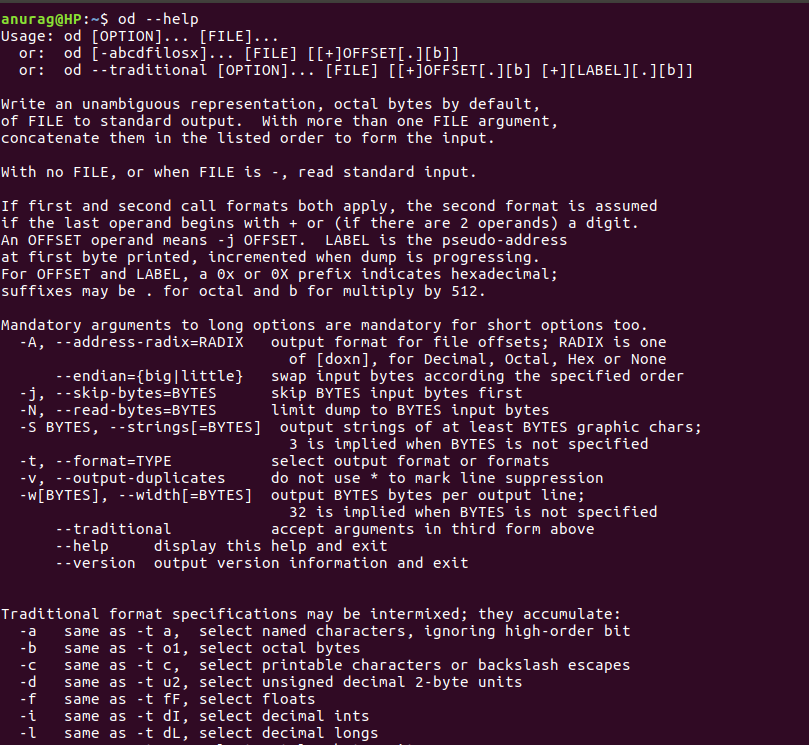16. –version Option :It displays version information.

SYNTAX :

```\$ \$ od --version
```

OUTPUT :